Two- and Three-Force Members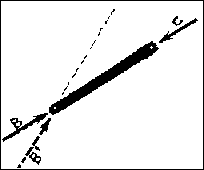There are many types of structural elements. It was seen in an earlier lecture that the support condition has a significant influence on the behavior of the specific element. It is advantagous to identify certain types of structural elements which have distinct characteristics. If an element has pins or hinge supports at both ends and carries no load in-between, it is called a two-force member. These elements can only have two forces acting upon them at their hinges. If only two forces act on a body that is in equilibrium, then they must be equal in magnitude, co-linear and opposite in sense. This is known as the two-force principle. The two-force principle applies to ANY member or structure that has only two forces acting on it. This is easily determined by simply counting the number of places where forces act on that member. (REMEMBER: reactions are considered to be forces!) If they act in two places, it is a two-force member.

One of the unique aspects of these members is the fact that the line of action of the resultants of the forces acting on the two ends of the member MUST pass along the center line of the structural element. If they did not, the element would not be in equilibrium! Thus, even if a loading exists at either end that consists of only one of the components (i.e. Fx or y), the resultant of all of the forces acting on the two-force member passes through the center line of the member.

Most, but not all, two-force members are straight. Straight elements are usually subjected to either tension or compression. Those members of other geometries will have bending across (or inside) their section in addition to tension or compression, but the two-force principle still applies. There are NO EXCEPTIONS!!!

Some common examples of two-force members are columns, struts, hangers, braces, pinned truss elements, chains, and cable-stayed suspension systems. What are some others?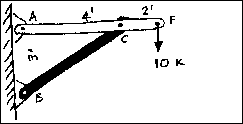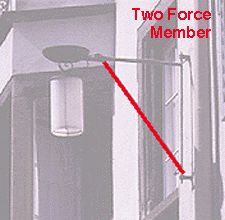Let us examine the simple system shown. It is a simplification of the lamp indicated. The load at point F is the hanging lamp. All of the joints are considered to be pinned. If member BC is isolated, it can be seen that it has forces acting at only points C and B. This means that it is a two-force member. The line of action of the force at point C must also pass through point B; similarly, a force at point B must also pass through point C. If the force at B did not pass through point C (B' in the diagram), the force would cause a moment about point C and equilibrium would not be possible. Because the two forces are equal in magnitude, co-linear and opposite in sense, two-force members act only in pure tension or pure compression. Supports such as cables tend to work well as two force members.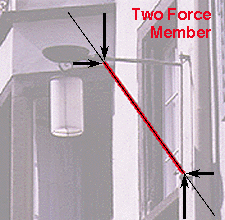If three non-parallel forces act on a body in equilibrium, it is known as a three-force member. The three forces interact with the structural element in a very specific manner in order to maintain equilibrium. If a three-force member is in equilibrium and the forces are not parallel, they must be concurrent. Therefore, the lines of action of all three forces acting on such a member must intersect at a common point; any single force is therfore the equilibrant of the other two forces. A three-force member is often an element which has a single load and two reactions. These members usually have forces which cause bending and sometimes additional tension and compression. The most common example of a three-force member is a simple beam.

The lamp example also has a three-force member as part of it's structural system.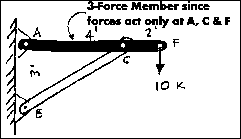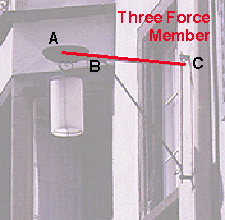If one isolates member AF in the pin connected frame to the right, one sees that it has forces acting at three points: A, C, and F. The free body diagram of the system can be seen in the diagram below. The magnitude and the line of action of the force at F, 10 Kips, is known. The line of action of the force at point C is known because it must be equal and opposite to the force C of the two-force member CB. The line of action of the forces at point F and point C intersect at X. The line of action of the force at point A must also go through points A and X. (Why is this?)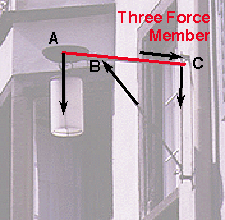The lines of action of the reactions at points A and C have now been determined. The problem of establishing their sense and magnitude remains. The sense of these forces can be established intuitively in this example, but this is not always the case. The Three Force Principle, demonstrated in a step-by-step manner, will show how simple it is to establish both the sense and the magnitude of the reactions of a system of three forces: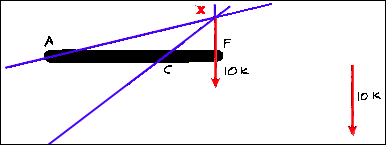1. Draw the load vector to some convenient scale.
2. Select either of the other forces and draw a line parallel to that force through the head of the load vector.
3. Through the tail of the load vector, draw a line parallel to the remaining force. (If these lines are extended far enough in each direction they form a closed polygon).

4.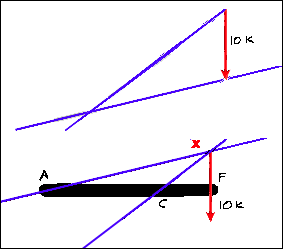5. Apply arrows on the vectors so that they are now connected head-to-tail. The force polygon is now complete; the arrows show the sense and the vectors can be scaled to determine the magnitude.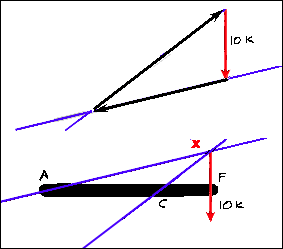Had it been assumed that the line of action of the reaction force going through point A had taken a direction other than through point X, the system would not be a concurrent force system. Although it could be in force equilibrium, it would not be in moment equilibrium because the summation of the moments about ANY point would no longer be zero. This can be seen below.

The following is an example of the resolution of the support force for the simple lamp system.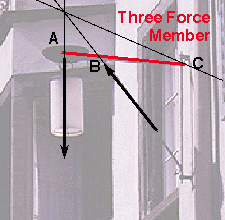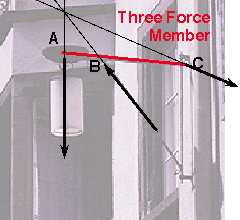The third force vector is drawn along the line of action passing through the second support. Now the sense and direction are known. If the diagram was drawn to scale, the magnitude of all of the forces could be simply determined using any graphical method of force resolution.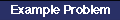Three-Force Members

hmmm.....

### Additional Reading

Schaeffer, R.E. Elementary Structures for Architects and Builders. Chapter 2.
Schodek, Daniel. Structures, Second Edition. pp 41 - 44.

Copyright © 1995, 1996 by Chris H. Luebkeman and Donald Peting
Copyright © 1997 by Chris H. Luebkeman